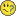# New to QlikView

Discussion board where members can get started with QlikView.

Announcements
Make your voice heard! Participate in the 2020 Wisdom of Crowds® Survey. BEGIN SURVEY
HighlightedNot applicable

Hi,

I am new to Qlikview and am trying to restrict the results of an expression using a Variable \$(vSales Gross) to only show values from the previous day.

I have tried =({<[Date]={'\$(=Date(today()-1))'}>} \$(vSales Gross))

Any help would be greatly appreciated

Tags (3)
1 Solution

Accepted Solutions
Highlighted
Esteemed Contributor III

sum({<[Date]={'\$(=Date(today()-1))'}>} [AGG Sales])

+sum({<[Date]={'\$(=Date(today()-1))'}>}[IB Sales])

+sum({<[Date]={'\$(=Date(today()-1))'}>}[OB Sales])

+sum({<[Date]={'\$(=Date(today()-1))'}>}[WEB Sales])

10 Replies
HighlightedMVP & Luminary

Try  =sum({<[Date]={'\$(=Date(today()-1))'}>} \$(vSales Gross))

talk is cheap, supply exceeds demand
Highlighted
Esteemed Contributor III

We have no idea what's inside that \$(vSales Gross)

It could be another sum() there.  In this case the set must be inside this variable.MVP & Luminary

True, we also have no idea if the Date field contains date values or text values or any values at all.

talk is cheap, supply exceeds demand
HighlightedNot applicable

Hi Tim,

Try below expression.

sum({<Date={'\$(=Date(today()-1))'}>} \$(vSales Gross))

Thanks,

krish

HighlightedNot applicable

try

Only({<[Date]={'\$(=Date(today()-1))'}>} \$(vSales Gross))

HighlightedNot applicable

Hi,

Thanks. The Date field is date values and the Variable does contain Sum().

Tim

Highlighted
Esteemed Contributor III

That means that the set should be inside the variable.  But you apparently use it somewhere else so can't change it.  In this case don't use it for the "yesterday" calculation.  Use expression per Gysbert but instead of the variable use field to sum.

If it is more complex than that, tell us what is the variable definition.

HighlightedNot applicable

Thanks,

The Variable definition is sum([AGG Sales])+sum([IB Sales])+sum([OB Sales])+sum([WEB Sales])

Tim

Highlighted
Esteemed Contributor III